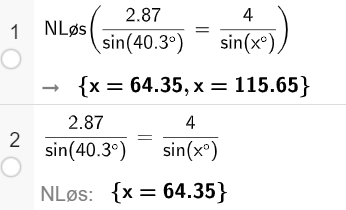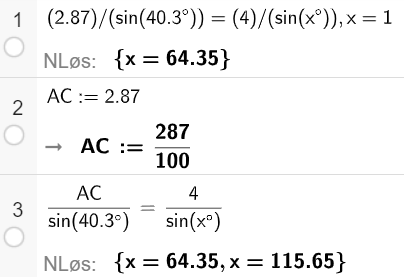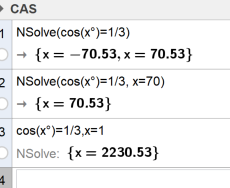# NSolve cos(x°) inconsistency between NSolve button and NSolve command

rn shared this problem 2 years ago
Not a Problem

CAS: Using the graphical NSolve button produces another result than the NSolve command.

Equation: cos(x°)=1/3

Result with NSolve button: {x=2230.53}

Result with Nsolve(cos(x°)=1/3): {x=-70.53,x=70.53}

Version: 6.0.631.0-offline (09 March 2021). Norwegian language.

Steps to reproduce:

1. Enter cos(x°)=1/3. Click NSolve button.

Result: {x=2230.53}. Step 1 in attached picture.

2. Click the line with cos(x°)=1/3. Press Enter.

Result: {x=-70.53,x=70.53}

3. Enter NLøs(cos(x°)=1/3). Press Enter.

Result: {x=-70.53,x=70.53}

Two problems:

Expected NSolve button and NSolve command to give the same small angle(s).

Expected pressing Enter on an existing line to not produce a similar but different result, but either showing the equation, or evaluating in the same way as the first time the equation was entered.1

Possibly a variant of the same problem? (NLøs is norwegian for NSolve)1

When AC was set to the value 2.87, the NSolve button gives both solutions, but not in line 1 when 2.87 was entered as a number:Also very strange: Line 1 changed from nicely formatted fractions to slashes and parentheses after evaluating line 3 with the NSolve button...1

The button has to guess between these syntaxes - if it doesn't give the "right" one then you'll need to enter it manually

```NSolve(cos(x°)=1/3)
NSolve(cos(x°)=1/3, x=70)```1

Both these give the same answer: {x=-70.53,x=70.53}

The problem is that the button gives another answer: {x=2230.53}1

You can edit the "x=1" (or remove it)1

I think I see what you mean with the guessing of the two kinds of syntaxes. Has it to do with the NSolve user guide comment "For non-polynomials you should always specify a starting value"? But anyway I think it is inconsistent. Why does the button have to guess, when NSolve does not?

When I write

`cos(x°)=1/3`
and click the button, I would expect the same answer as I get when I write

`NSolve(cos(x°)=1/3)`

Why does not these two "methods" give the same answer? It is natural (for me at least) to expect NSolve without starting value to give the same result as clicking the NSolve-button without a starting value. There is probably something here that I do not have full knowledge, but when NSolve without starting value does a great job of finding a sensible solution, it would be very nice if the button would do the same great job.

PS: The x=1 does not appear after clicking the button (as it did in previous versions, maybe?).1

```For non-polynomials you should always specify a starting value
```

Yes, exactly. When dealing with numerical solving I'm afraid there can be no guarantees - use Solve() if you need that1

```The x=1 does not appear after clicking the button
```

Sorry, that's in Classic 5 only